# Sorting Algorithms Sorting Sorting is a process that

• Slides: 57Sorting Algorithms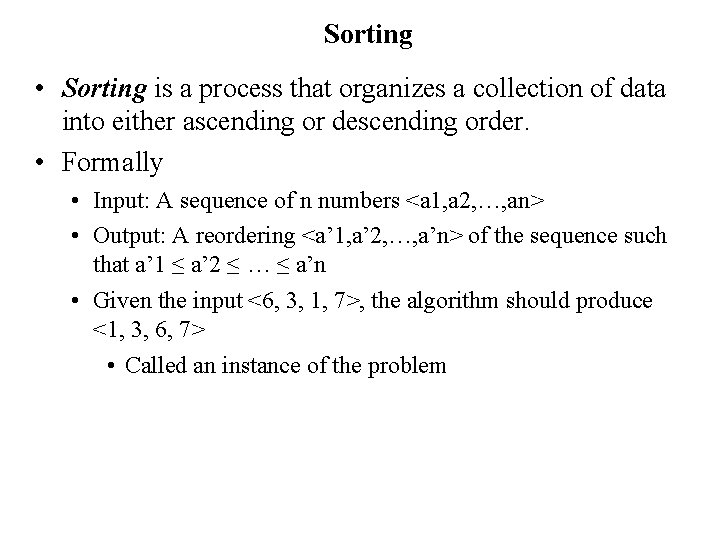Sorting • Sorting is a process that organizes a collection of data into either ascending or descending order. • Formally • Input: A sequence of n numbers <a 1, a 2, …, an> • Output: A reordering <a’ 1, a’ 2, …, a’n> of the sequence such that a’ 1 ≤ a’ 2 ≤ … ≤ a’n • Given the input <6, 3, 1, 7>, the algorithm should produce <1, 3, 6, 7> • Called an instance of the problem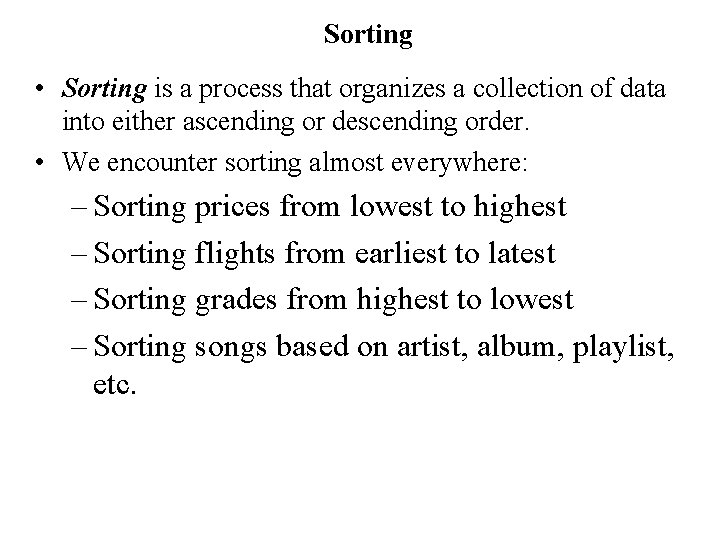Sorting • Sorting is a process that organizes a collection of data into either ascending or descending order. • We encounter sorting almost everywhere: – Sorting prices from lowest to highest – Sorting flights from earliest to latest – Sorting grades from highest to lowest – Sorting songs based on artist, album, playlist, etc.Sorting Algorithms • There are many sorting algorithms (as of 27. 10. 2014 there are 44 Wikipedia entries) • In this class we will learn: – Selection Sort – Insertion Sort – Bubble Sort – Merge Sort – Quick Sort – Bogo sort and Sleep sort are some bad/slow algorithms • These are among the most fundamental sorting algorithms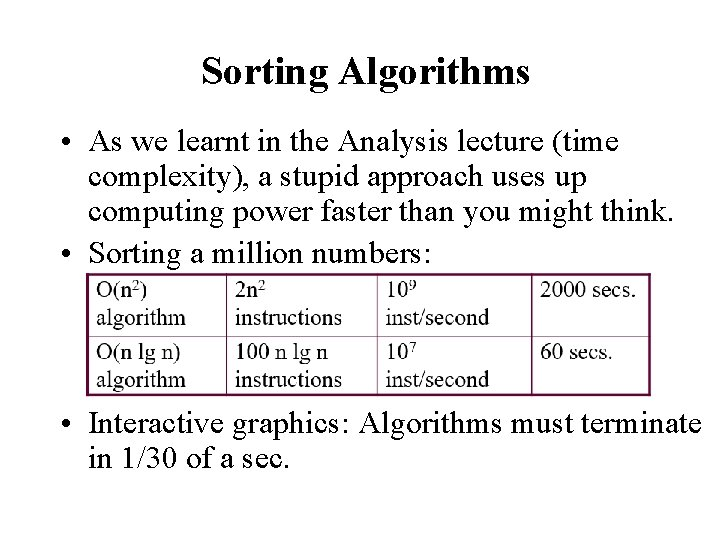Sorting Algorithms • As we learnt in the Analysis lecture (time complexity), a stupid approach uses up computing power faster than you might think. • Sorting a million numbers: • Interactive graphics: Algorithms must terminate in 1/30 of a sec.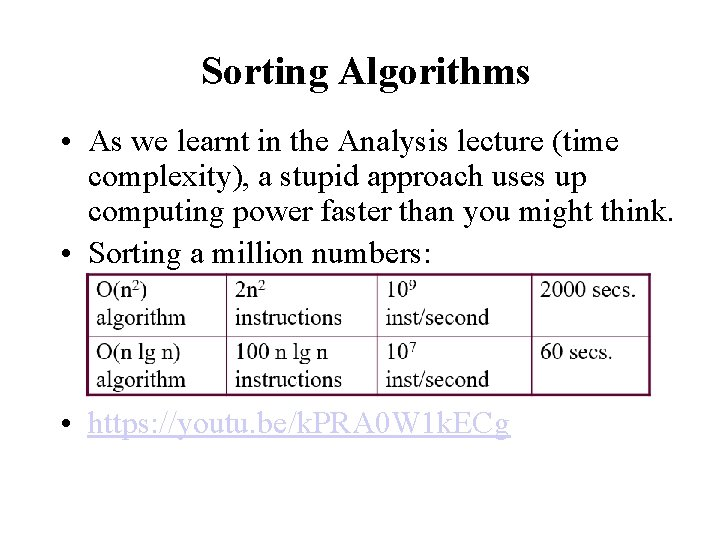Sorting Algorithms • As we learnt in the Analysis lecture (time complexity), a stupid approach uses up computing power faster than you might think. • Sorting a million numbers: • https: //youtu. be/k. PRA 0 W 1 k. ECg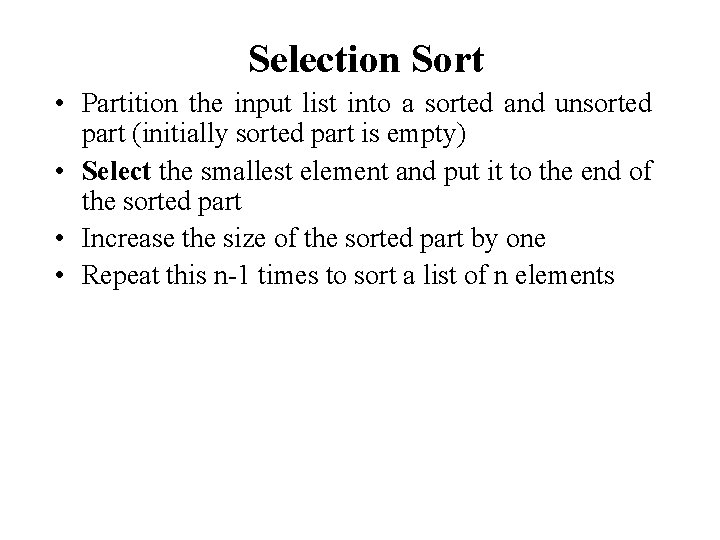Selection Sort • Partition the input list into a sorted and unsorted part (initially sorted part is empty) • Select the smallest element and put it to the end of the sorted part • Increase the size of the sorted part by one • Repeat this n-1 times to sort a list of n elementsSorted Unsorted 23 78 45 8 32 56 Original List 8 78 45 23 32 56 After pass 1 8 23 45 78 32 56 After pass 2 8 8 8 23 23 23 32 32 32 78 45 45 45 78 56 56 56 78 After pass 3 After pass 4 After pass 5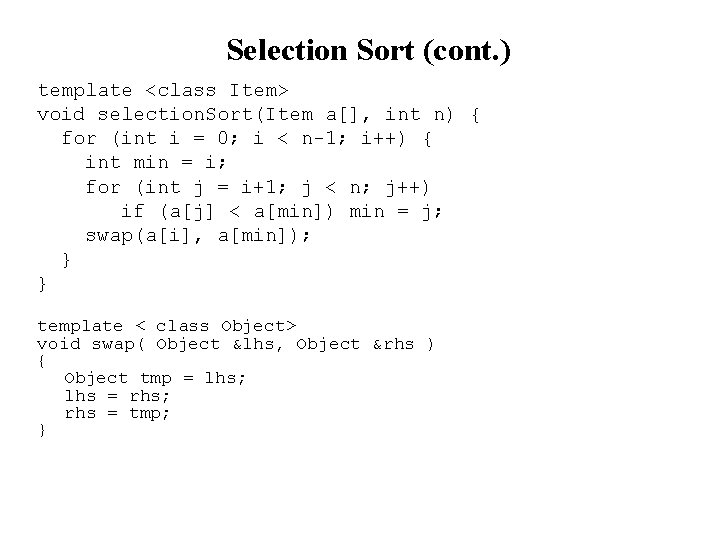Selection Sort (cont. ) template <class Item> void selection. Sort(Item a[], int n) { for (int i = 0; i < n-1; i++) { int min = i; for (int j = i+1; j < n; j++) if (a[j] < a[min]) min = j; swap(a[i], a[min]); } } template < class Object> void swap( Object &lhs, Object &rhs ) { Object tmp = lhs; lhs = rhs; rhs = tmp; }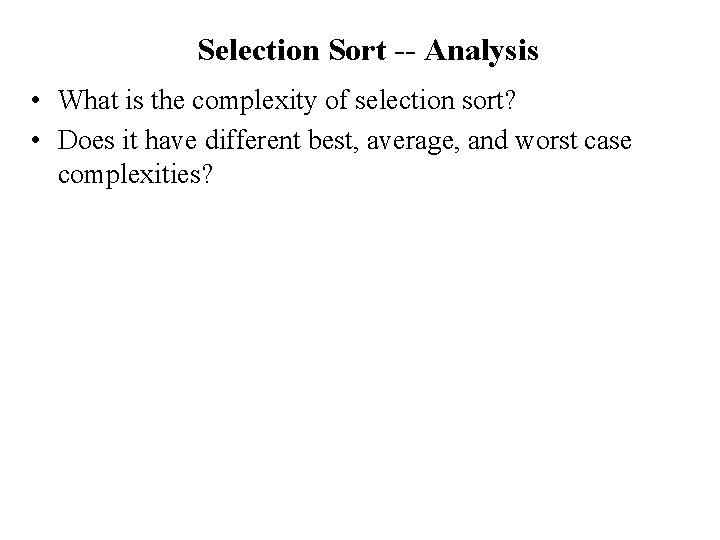Selection Sort -- Analysis • What is the complexity of selection sort? • Does it have different best, average, and worst case complexities?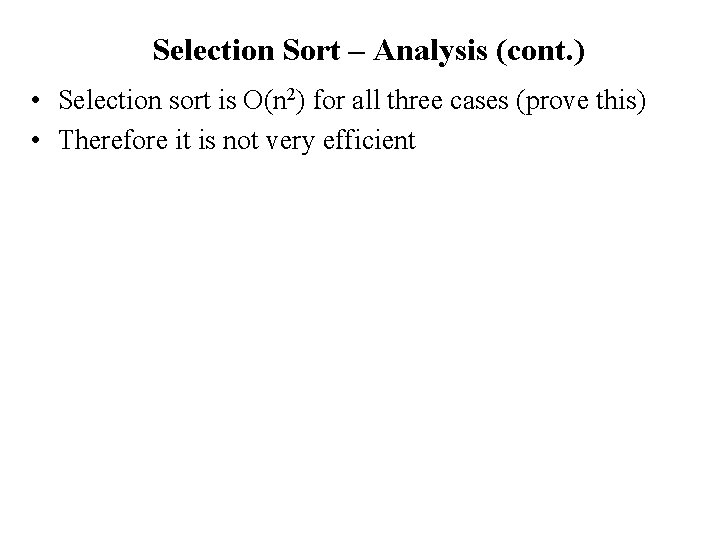Selection Sort – Analysis (cont. ) • Selection sort is O(n 2) for all three cases (prove this) • Therefore it is not very efficientInsertion Sort • Insertion sort is a simple sorting algorithm that is appropriate for small inputs. – Most common sorting technique used by card players. • Again, the list is divided into two parts: sorted and unsorted. • In each pass, the first element of the unsorted part is picked up, transferred to the sorted sublist, and inserted at the appropriate place. • A list of n elements will take at most n-1 passes to sort the data.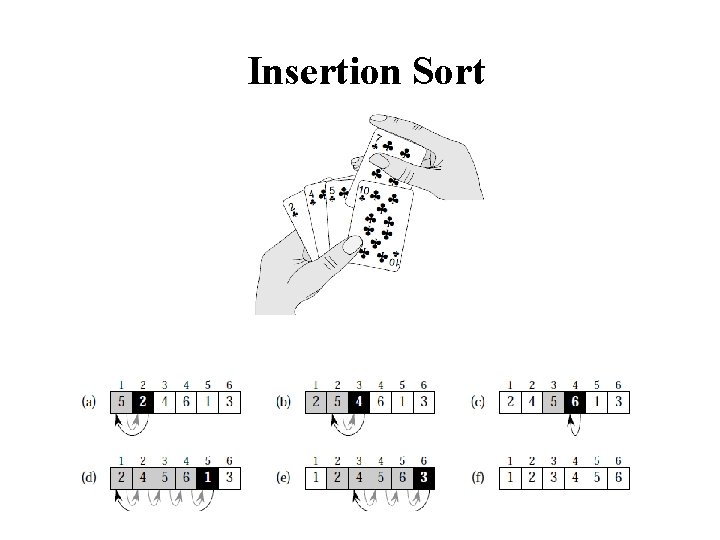Insertion Sort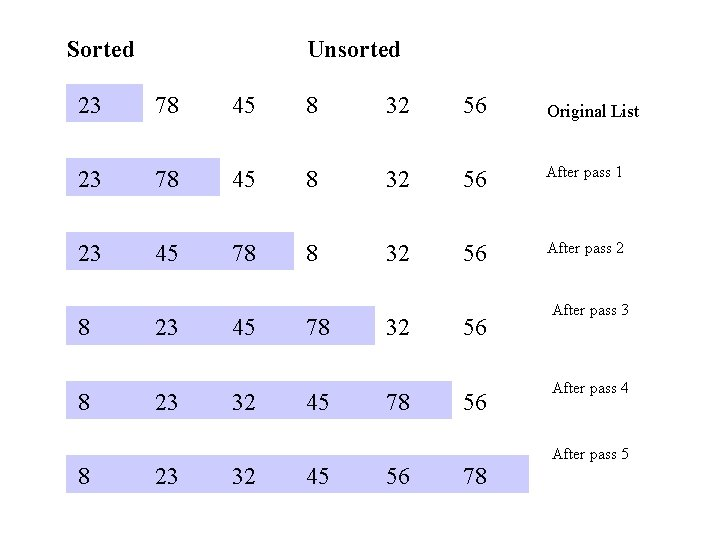Sorted Unsorted 23 78 45 8 32 56 Original List 23 78 45 8 32 56 After pass 1 23 45 78 8 32 56 After pass 2 8 8 8 23 23 23 45 32 32 78 45 45 32 78 56 56 56 78 After pass 3 After pass 4 After pass 5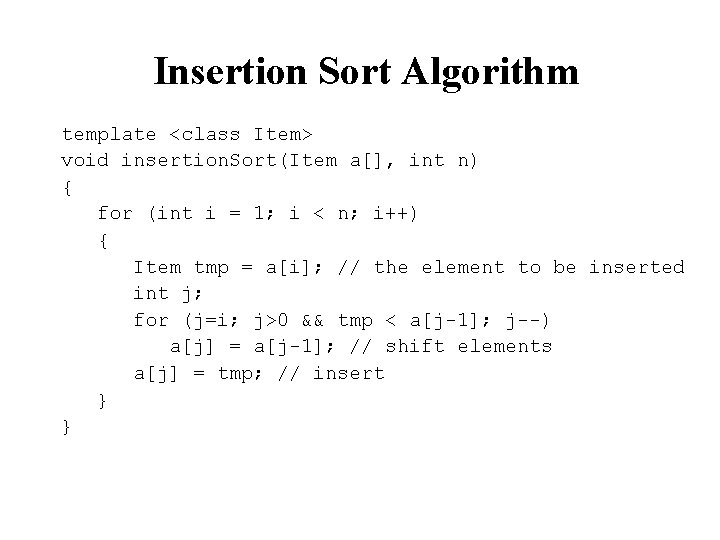Insertion Sort Algorithm template <class Item> void insertion. Sort(Item a[], int n) { for (int i = 1; i < n; i++) { Item tmp = a[i]; // the element to be inserted int j; for (j=i; j>0 && tmp < a[j-1]; j--) a[j] = a[j-1]; // shift elements a[j] = tmp; // insert } }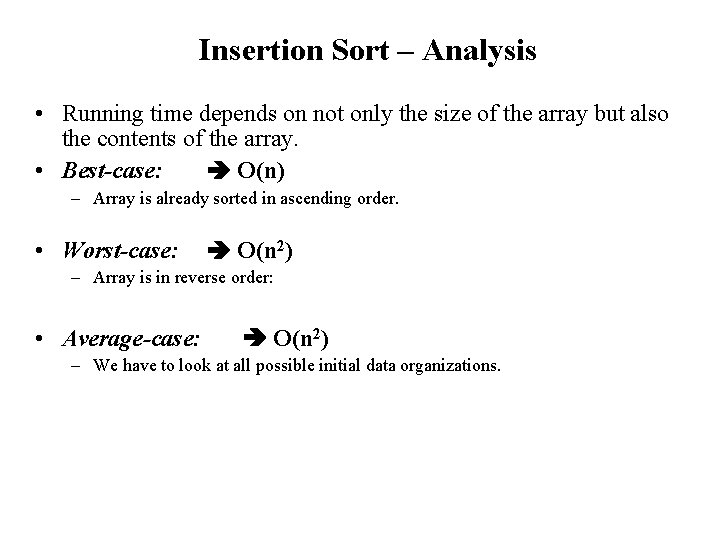Insertion Sort – Analysis • Running time depends on not only the size of the array but also the contents of the array. • Best-case: O(n) – Array is already sorted in ascending order. • Worst-case: O(n 2) – Array is in reverse order: • Average-case: O(n 2) – We have to look at all possible initial data organizations.Analysis of insertion sort • Which running time will be used to characterize this algorithm? – Best, worst or average? • Worst: – Longest running time (this is the upper limit for the algorithm) – It is guaranteed that the algorithm will not be worse than this. • Sometimes we are interested in average case. But there are some problems with the average case. – It is difficult to figure out the average case. i. e. what is average input? – Are we going to assume all possible inputs are equally likely? – In fact for most algorithms average case is same as the worst case.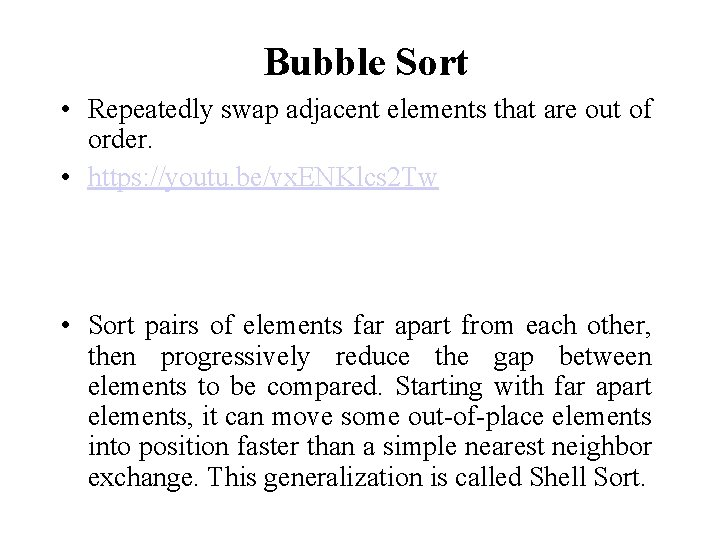Bubble Sort • Repeatedly swap adjacent elements that are out of order. • https: //youtu. be/vx. ENKlcs 2 Tw • Sort pairs of elements far apart from each other, then progressively reduce the gap between elements to be compared. Starting with far apart elements, it can move some out-of-place elements into position faster than a simple nearest neighbor exchange. This generalization is called Shell Sort.Bubble Sort 23 78 45 8 32 56 23 45 78 8 32 56 23 45 8 78 32 56 23 45 8 32 78 56 23 45 8 32 56 78 23 8 45 32 56 78 8 23 32 45 56 78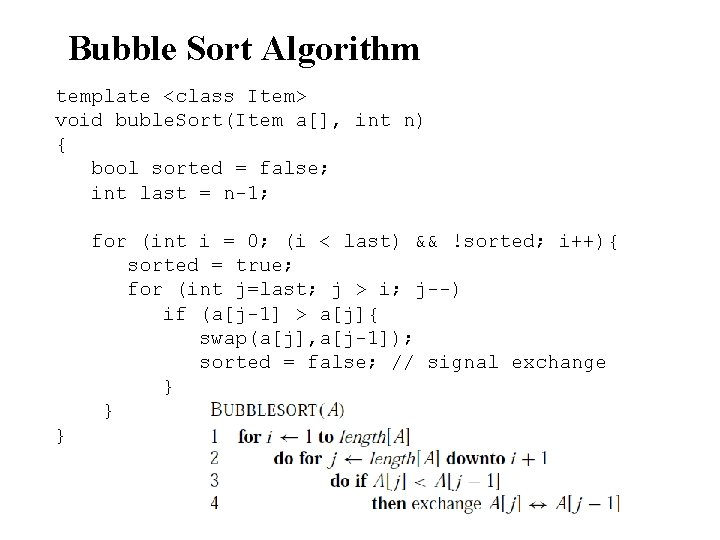Bubble Sort Algorithm template <class Item> void buble. Sort(Item a[], int n) { bool sorted = false; int last = n-1; for (int i = 0; (i < last) && !sorted; i++){ sorted = true; for (int j=last; j > i; j--) if (a[j-1] > a[j]{ swap(a[j], a[j-1]); sorted = false; // signal exchange } } }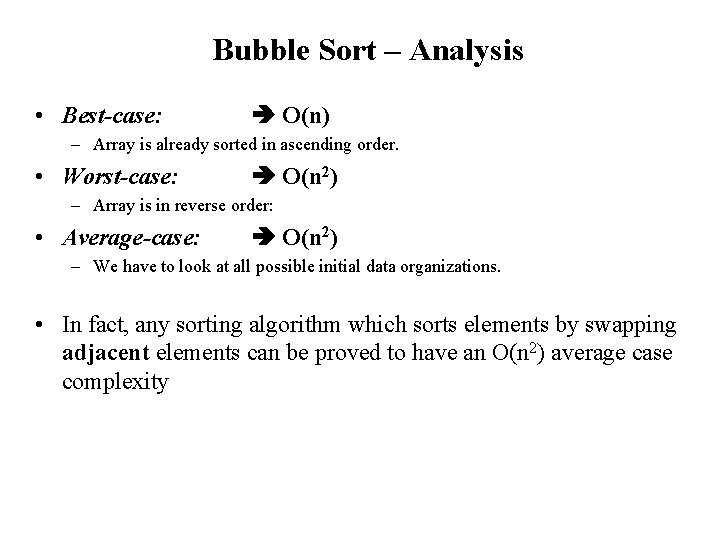Bubble Sort – Analysis • Best-case: O(n) – Array is already sorted in ascending order. • Worst-case: O(n 2) – Array is in reverse order: • Average-case: O(n 2) – We have to look at all possible initial data organizations. • In fact, any sorting algorithm which sorts elements by swapping adjacent elements can be proved to have an O(n 2) average case complexity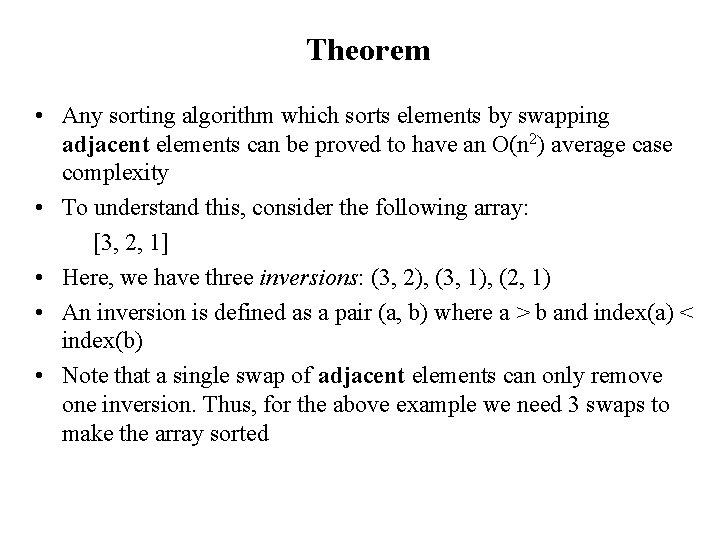Theorem • Any sorting algorithm which sorts elements by swapping adjacent elements can be proved to have an O(n 2) average case complexity • To understand this, consider the following array: • [3, 2, 1] • Here, we have three inversions: (3, 2), (3, 1), (2, 1) • An inversion is defined as a pair (a, b) where a > b and index(a) < index(b) • Note that a single swap of adjacent elements can only remove one inversion. Thus, for the above example we need 3 swaps to make the array sorted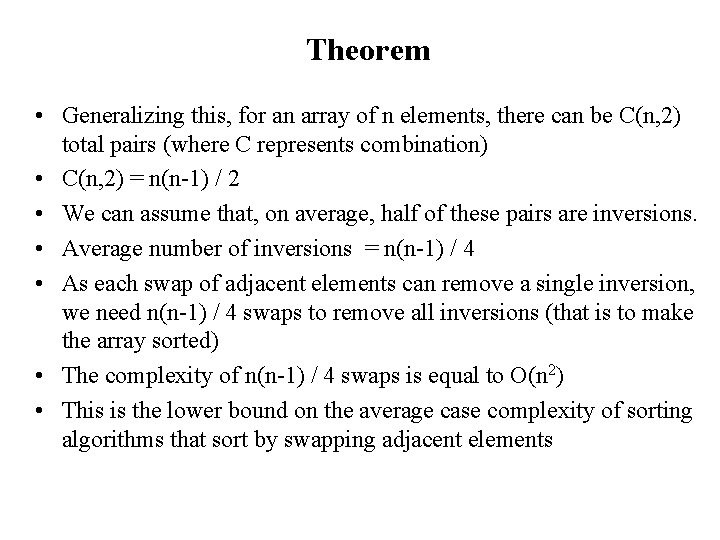Theorem • Generalizing this, for an array of n elements, there can be C(n, 2) total pairs (where C represents combination) • C(n, 2) = n(n-1) / 2 • We can assume that, on average, half of these pairs are inversions. • Average number of inversions = n(n-1) / 4 • As each swap of adjacent elements can remove a single inversion, we need n(n-1) / 4 swaps to remove all inversions (that is to make the array sorted) • The complexity of n(n-1) / 4 swaps is equal to O(n 2) • This is the lower bound on the average case complexity of sorting algorithms that sort by swapping adjacent elements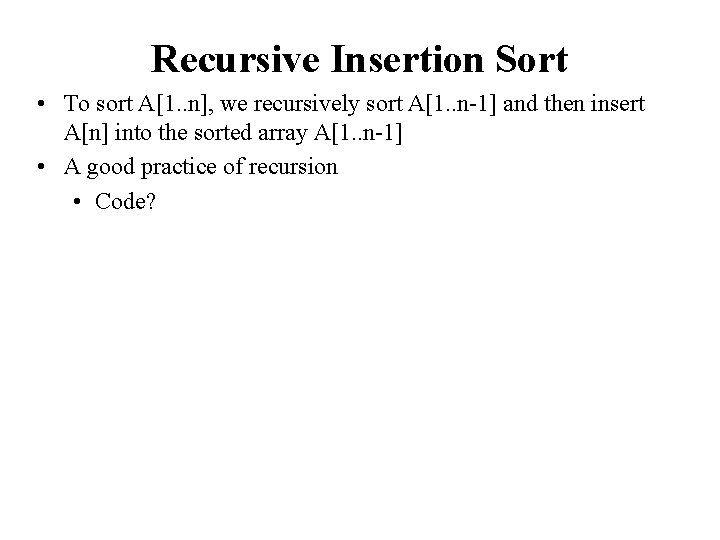Recursive Insertion Sort • To sort A[1. . n], we recursively sort A[1. . n-1] and then insert A[n] into the sorted array A[1. . n-1] • A good practice of recursion • Code?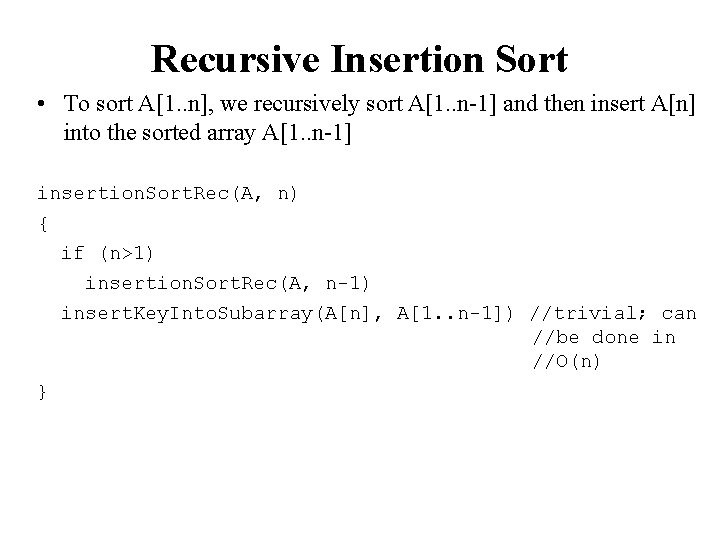Recursive Insertion Sort • To sort A[1. . n], we recursively sort A[1. . n-1] and then insert A[n] into the sorted array A[1. . n-1] insertion. Sort. Rec(A, n) { if (n>1) insertion. Sort. Rec(A, n-1) insert. Key. Into. Subarray(A[n], A[1. . n-1]) //trivial; can //be done in //O(n) }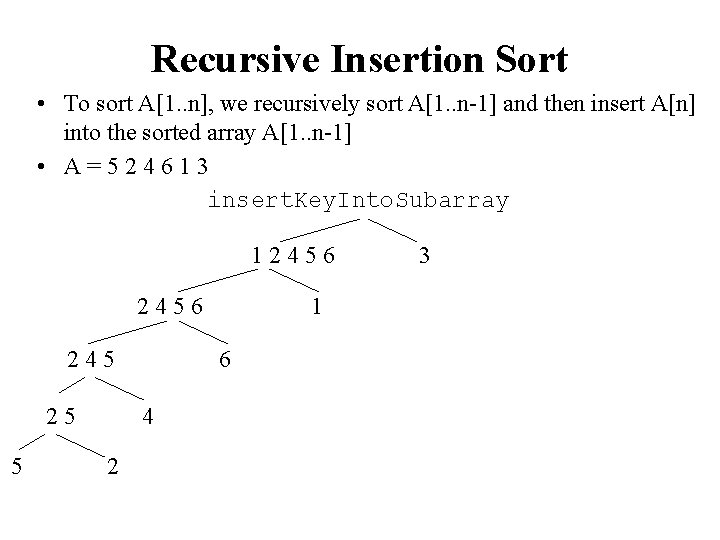Recursive Insertion Sort • To sort A[1. . n], we recursively sort A[1. . n-1] and then insert A[n] into the sorted array A[1. . n-1] • A=524613 insert. Key. Into. Subarray 12456 245 25 5 6 4 2 1 3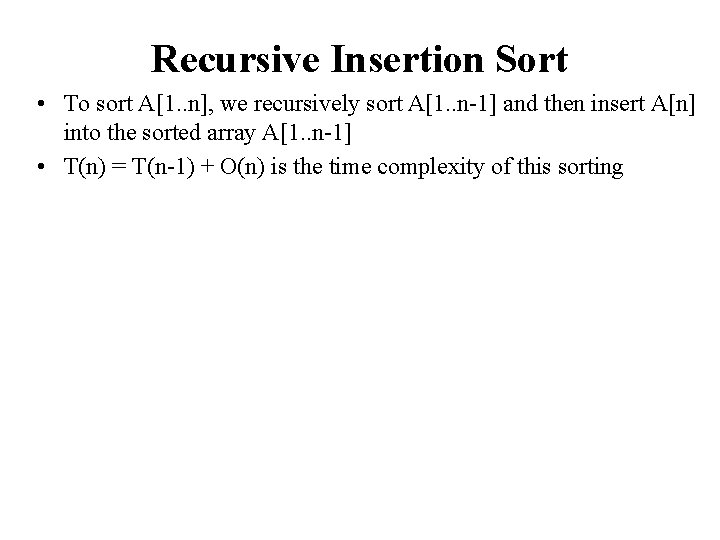Recursive Insertion Sort • To sort A[1. . n], we recursively sort A[1. . n-1] and then insert A[n] into the sorted array A[1. . n-1] • T(n) = T(n-1) + O(n) is the time complexity of this sortingRecursive Insertion Sort • To sort A[1. . n], we recursively sort A[1. . n-1] and then insert A[n] into the sorted array A[1. . n-1] • T(n) = T(n-1) + O(n) • Reduce the problem size to n-1 w/ O(n) extra work • Seems to be doing O(n) work n-1 times; so O(n 2) guess? • T(n) ≤ cn 2 //assume holds T(n) ≤ c(n-1)2 + dn = cn 2 -2 cn + c + dn = cn 2 –c(2 n -1) + dn ≤ cn 2 //because large values of c dominates d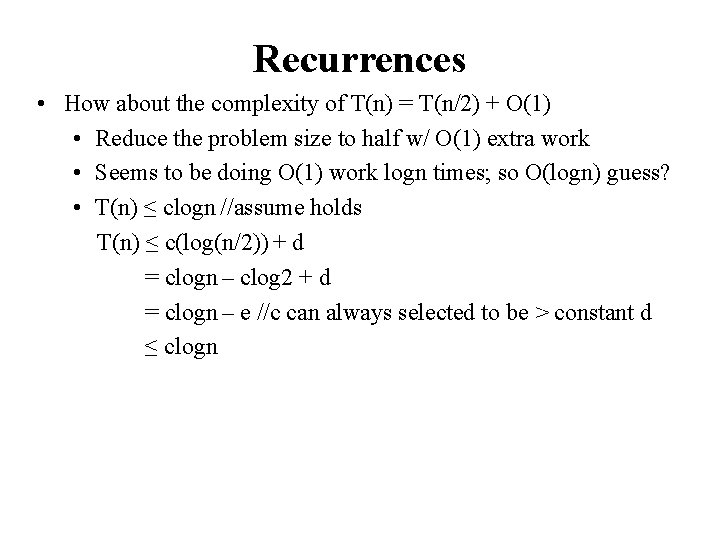Recurrences • How about the complexity of T(n) = T(n/2) + O(1) • Reduce the problem size to half w/ O(1) extra work • Seems to be doing O(1) work logn times; so O(logn) guess? • T(n) ≤ clogn //assume holds T(n) ≤ c(log(n/2)) + d = clogn – clog 2 + d = clogn – e //c can always selected to be > constant d ≤ clogn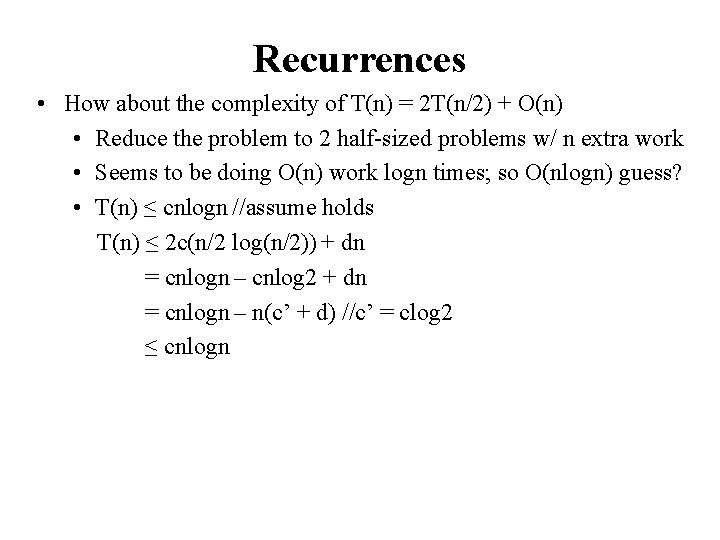Recurrences • How about the complexity of T(n) = 2 T(n/2) + O(n) • Reduce the problem to 2 half-sized problems w/ n extra work • Seems to be doing O(n) work logn times; so O(nlogn) guess? • T(n) ≤ cnlogn //assume holds T(n) ≤ 2 c(n/2 log(n/2)) + dn = cnlogn – cnlog 2 + dn = cnlogn – n(c’ + d) //c’ = clog 2 ≤ cnlogn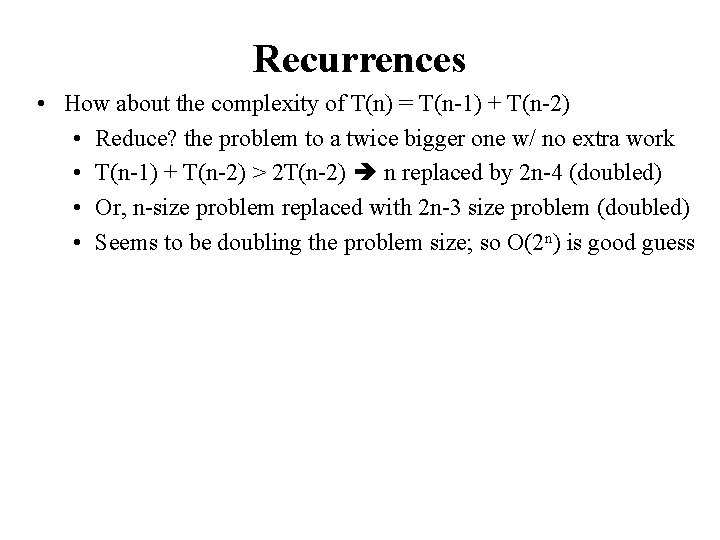Recurrences • How about the complexity of T(n) = T(n-1) + T(n-2) • Reduce? the problem to a twice bigger one w/ no extra work • T(n-1) + T(n-2) > 2 T(n-2) n replaced by 2 n-4 (doubled) • Or, n-size problem replaced with 2 n-3 size problem (doubled) • Seems to be doubling the problem size; so O(2 n) is good guess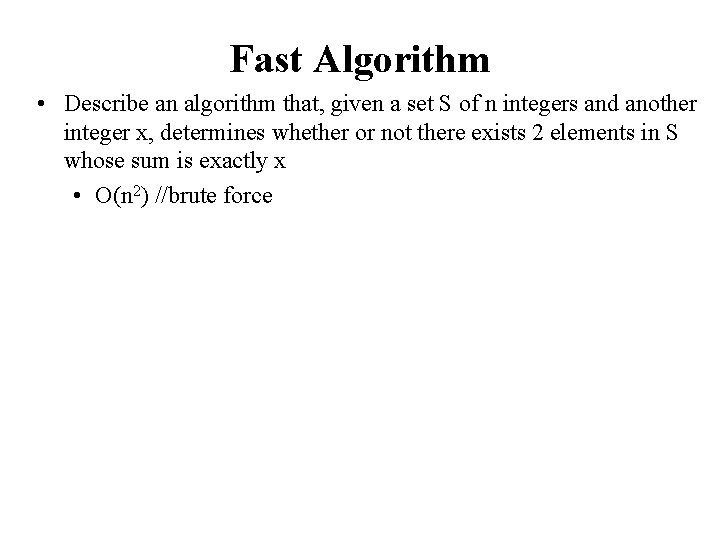Fast Algorithm • Describe an algorithm that, given a set S of n integers and another integer x, determines whether or not there exists 2 elements in S whose sum is exactly x • O(n 2) //brute force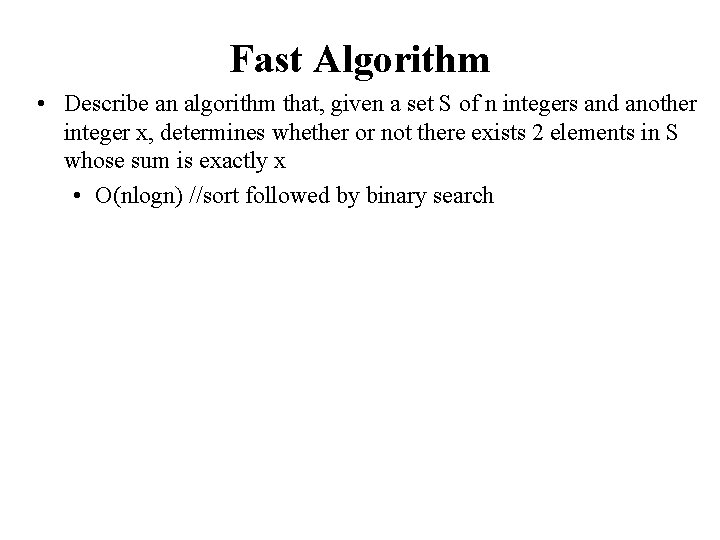Fast Algorithm • Describe an algorithm that, given a set S of n integers and another integer x, determines whether or not there exists 2 elements in S whose sum is exactly x • O(nlogn) //sort followed by binary search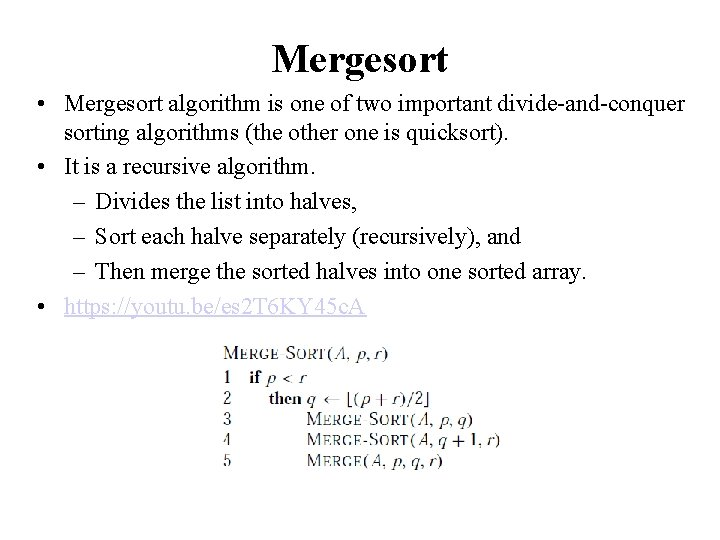Mergesort • Mergesort algorithm is one of two important divide-and-conquer sorting algorithms (the other one is quicksort). • It is a recursive algorithm. – Divides the list into halves, – Sort each halve separately (recursively), and – Then merge the sorted halves into one sorted array. • https: //youtu. be/es 2 T 6 KY 45 c. AMergesort - Example 6 3 9 divide 15472 6 3 9 1 5 4 7 2 divide 7 2 6 3 9 1 5 4 divide 6 3 9 merge 3 6 merge 1 5 4 merge 1 9 4 1 3 6 9 divide 7 merge 5 2 7 merge 2 4 5 7 merge 12345679 2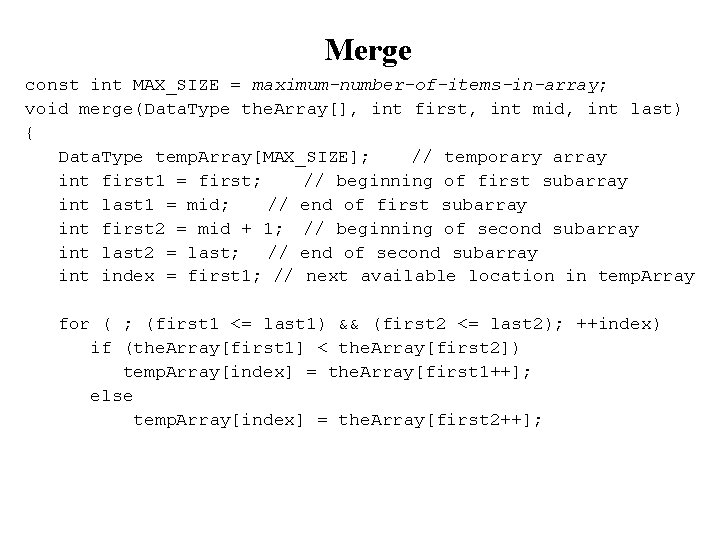Merge const int MAX_SIZE = maximum-number-of-items-in-array; void merge(Data. Type the. Array[], int first, int mid, int last) { Data. Type temp. Array[MAX_SIZE]; // temporary array int first 1 = first; // beginning of first subarray int last 1 = mid; // end of first subarray int first 2 = mid + 1; // beginning of second subarray int last 2 = last; // end of second subarray int index = first 1; // next available location in temp. Array for ( ; (first 1 <= last 1) && (first 2 <= last 2); ++index) if (the. Array[first 1] < the. Array[first 2]) temp. Array[index] = the. Array[first 1++]; else temp. Array[index] = the. Array[first 2++];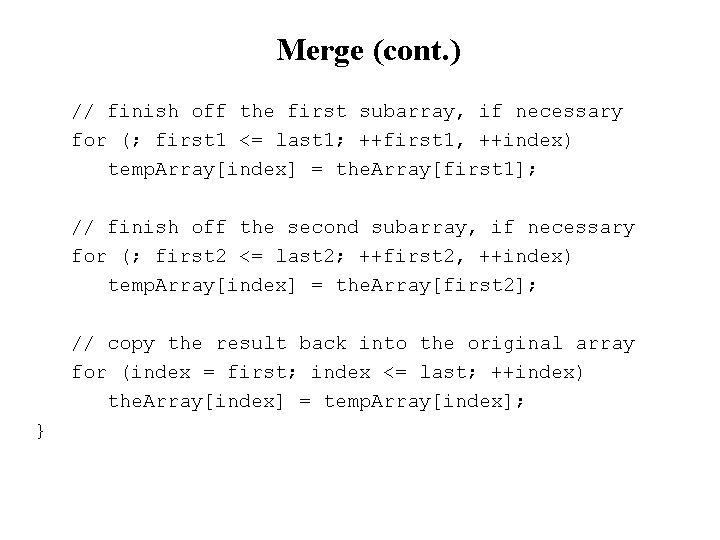Merge (cont. ) // finish off the first subarray, if necessary for (; first 1 <= last 1; ++first 1, ++index) temp. Array[index] = the. Array[first 1]; // finish off the second subarray, if necessary for (; first 2 <= last 2; ++first 2, ++index) temp. Array[index] = the. Array[first 2]; // copy the result back into the original array for (index = first; index <= last; ++index) the. Array[index] = temp. Array[index]; }Mergesort void mergesort(Data. Type the. Array[], int first, int last) { if (first < last) { // more than one item int mid = (first + last)/2; // index of midpoint mergesort(the. Array, first, mid); mergesort(the. Array, mid+1, last); // merge the two halves merge(the. Array, first, mid, last); } } // end mergesort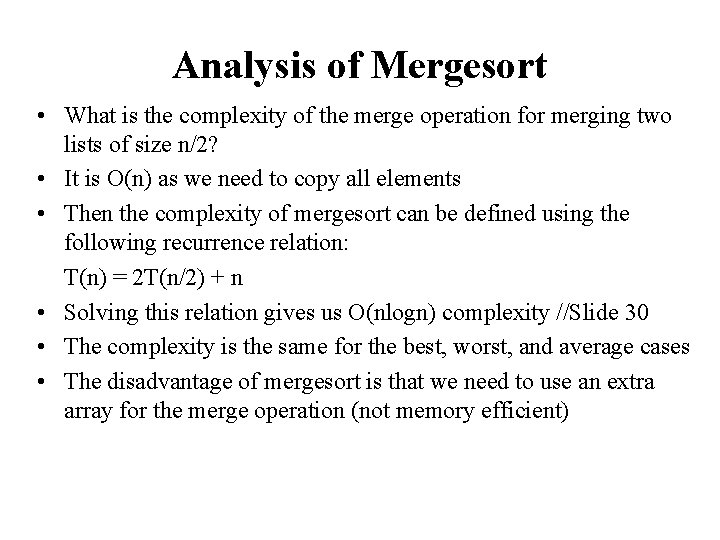Analysis of Mergesort • What is the complexity of the merge operation for merging two lists of size n/2? • It is O(n) as we need to copy all elements • Then the complexity of mergesort can be defined using the following recurrence relation: T(n) = 2 T(n/2) + n • Solving this relation gives us O(nlogn) complexity //Slide 30 • The complexity is the same for the best, worst, and average cases • The disadvantage of mergesort is that we need to use an extra array for the merge operation (not memory efficient)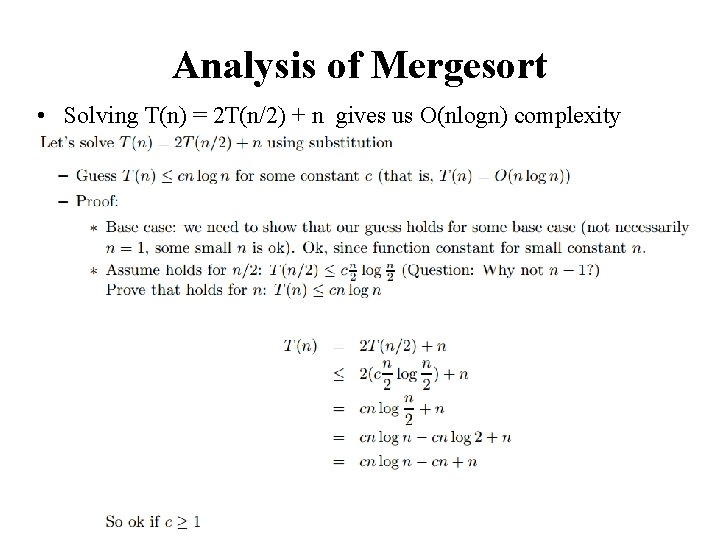Analysis of Mergesort • Solving T(n) = 2 T(n/2) + n gives us O(nlogn) complexity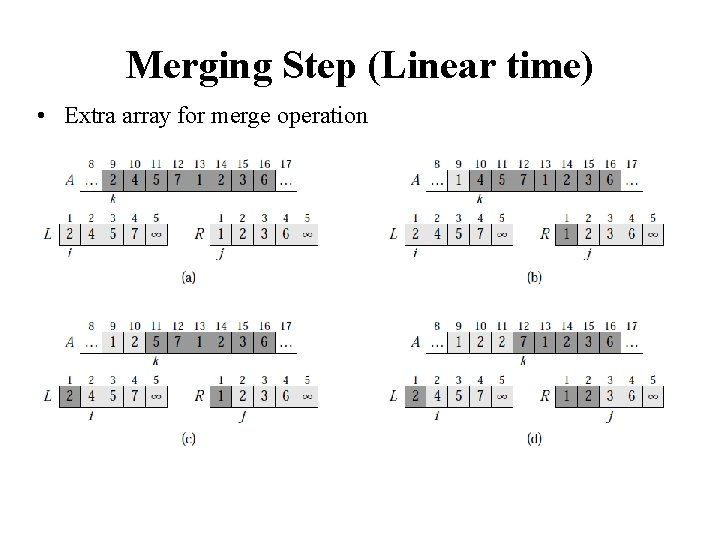Merging Step (Linear time) • Extra array for merge operation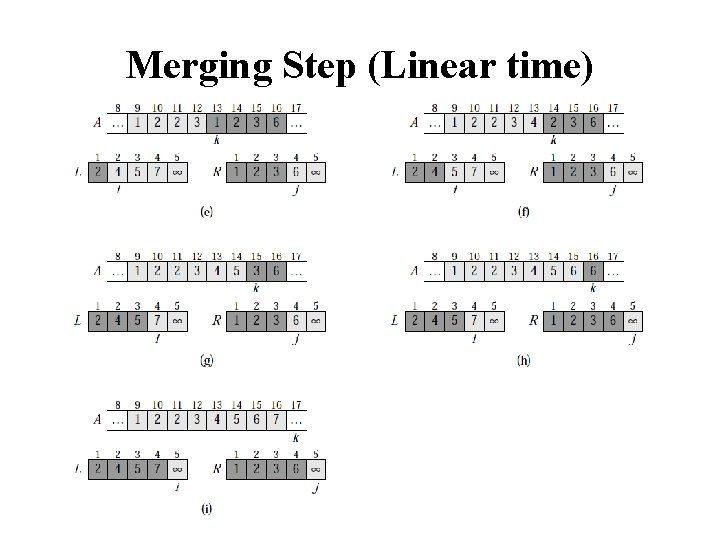Merging Step (Linear time)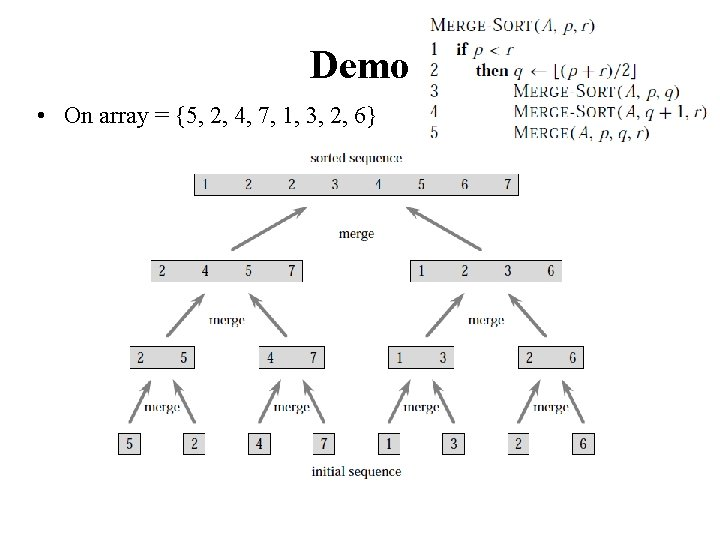Demo • On array = {5, 2, 4, 7, 1, 3, 2, 6}Quicksort • Like mergesort, quicksort is also based on the divide-and-conquer paradigm. • But it uses this technique in a somewhat opposite manner, as all the hard work is done before the recursive calls. • It works as follows: 1. First selects a pivot element, 2. Then it partitions an array into two parts (elements smaller than and greater than or equal to the pivot) 3. Then, it sorts the parts independently (recursively), 4. Finally, it combines the sorted subsequences by a simple concatenation. • https: //youtu. be/vx. ENKlcs 2 TwPartition • Partitioning places the pivot in its correct place position within the array. • Arranging the array elements around the pivot p generates two smaller sorting problems. – sort the left section of the array, and sort the right section of the array. – when these two smaller sorting problems are solved recursively, our bigger sorting problem is solved.Pivot Selection • Which array item should be selected as pivot? – Somehow we have to select a pivot, and we hope that we will get a good partitioning. – If the items in the array arranged randomly, we choose a pivot randomly. – We can choose the first or last element as the pivot (it may not give a good partitioning). – We can choose the middle element as the pivot – We can use a combination of the above to select the pivot (in each recursive call a different technique can be used)Partitioning Initial state of the array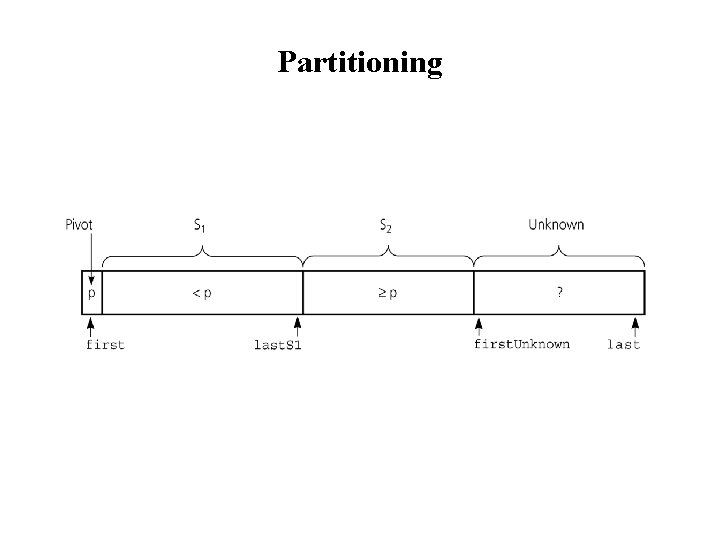PartitioningPartitioning Moving the. Array[first. Unknown] into S 1 by swapping it with the. Array[last. S 1+1] and by incrementing both last. S 1 and first. Unknown.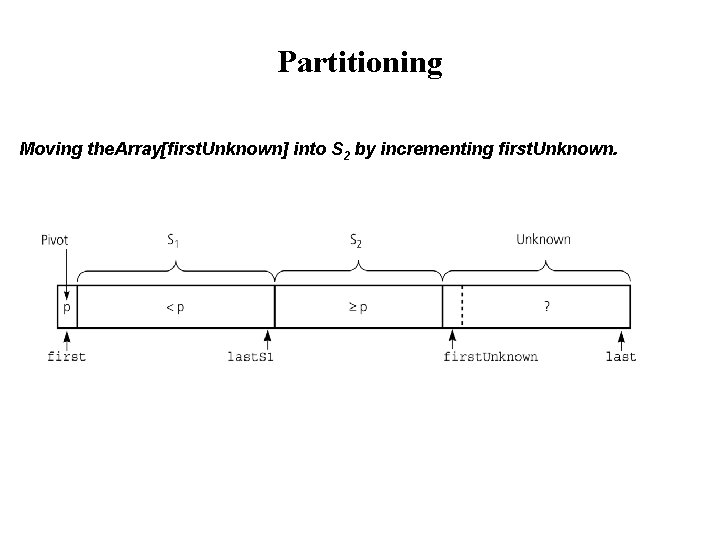Partitioning Moving the. Array[first. Unknown] into S 2 by incrementing first. Unknown.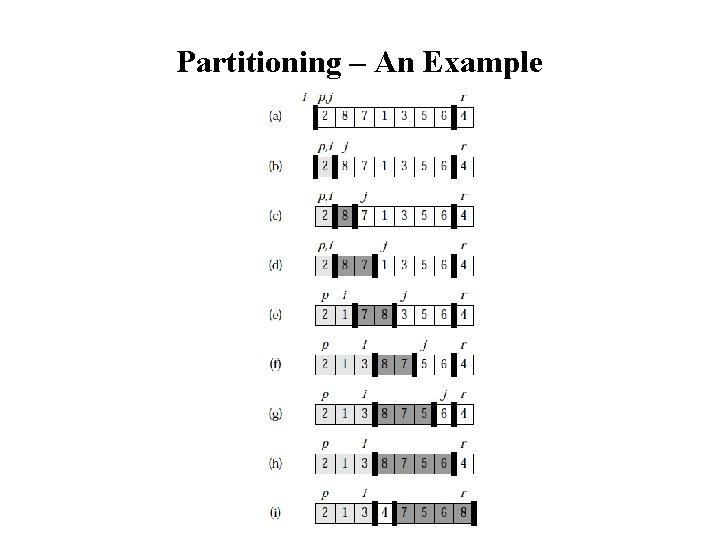Partitioning – An ExamplePartition Function template <class Data. Type> void partition(Data. Type the. Array[], int first, int last, int &pivot. Index) { int p. Index = choose. Pivot(the. Array, first, last); // put pivot at position first swap(the. Array[p. Index], the. Array[first]); Data. Type pivot = the. Array[first]; // copy pivot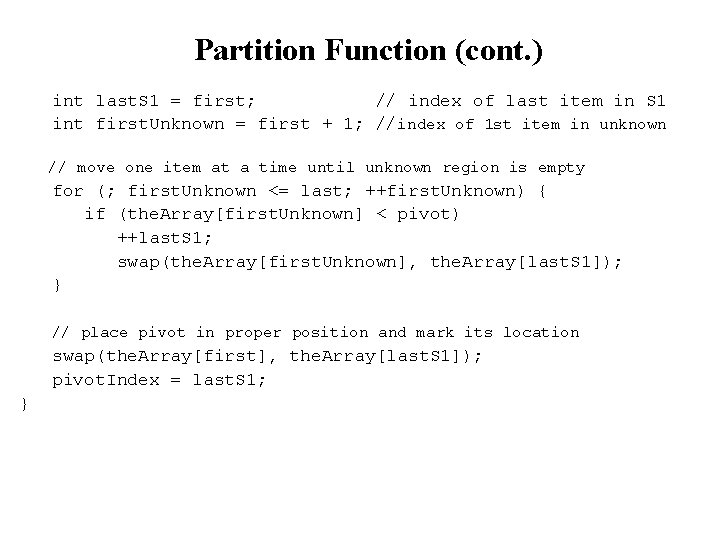Partition Function (cont. ) int last. S 1 = first; // index of last item in S 1 int first. Unknown = first + 1; //index of 1 st item in unknown // move one item at a time until unknown region is empty for (; first. Unknown <= last; ++first. Unknown) { if (the. Array[first. Unknown] < pivot) ++last. S 1; swap(the. Array[first. Unknown], the. Array[last. S 1]); } // place pivot in proper position and mark its location swap(the. Array[first], the. Array[last. S 1]); pivot. Index = last. S 1; }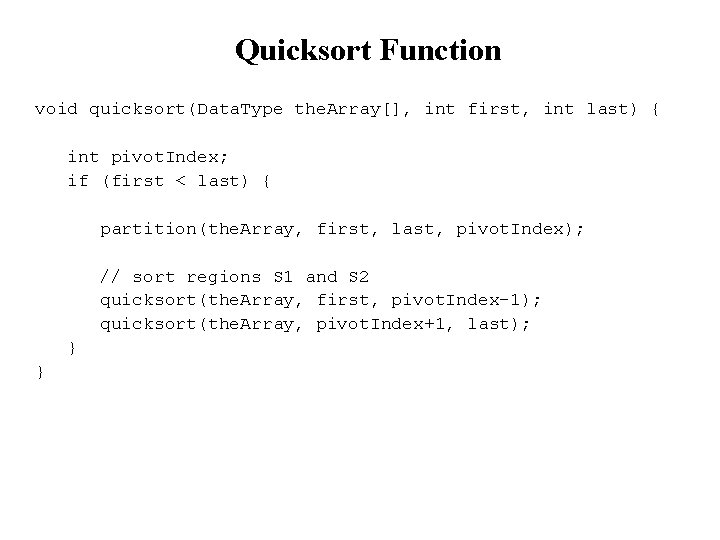Quicksort Function void quicksort(Data. Type the. Array[], int first, int last) { int pivot. Index; if (first < last) { partition(the. Array, first, last, pivot. Index); // sort regions S 1 and S 2 quicksort(the. Array, first, pivot. Index-1); quicksort(the. Array, pivot. Index+1, last); } }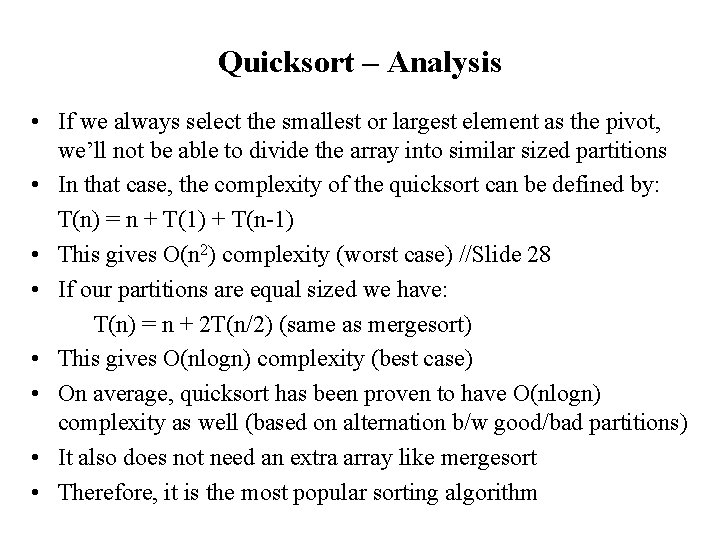Quicksort – Analysis • If we always select the smallest or largest element as the pivot, we’ll not be able to divide the array into similar sized partitions • In that case, the complexity of the quicksort can be defined by: T(n) = n + T(1) + T(n-1) • This gives O(n 2) complexity (worst case) //Slide 28 • If our partitions are equal sized we have: T(n) = n + 2 T(n/2) (same as mergesort) • This gives O(nlogn) complexity (best case) • On average, quicksort has been proven to have O(nlogn) complexity as well (based on alternation b/w good/bad partitions) • It also does not need an extra array like mergesort • Therefore, it is the most popular sorting algorithm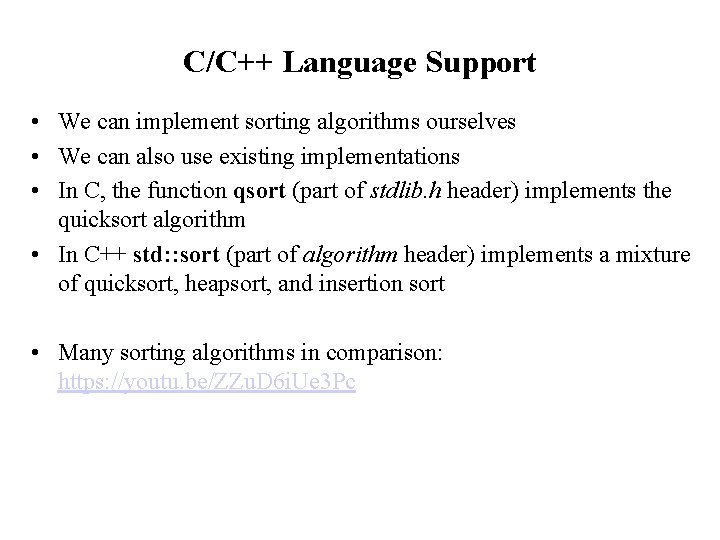C/C++ Language Support • We can implement sorting algorithms ourselves • We can also use existing implementations • In C, the function qsort (part of stdlib. h header) implements the quicksort algorithm • In C++ std: : sort (part of algorithm header) implements a mixture of quicksort, heapsort, and insertion sort • Many sorting algorithms in comparison: https: //youtu. be/ZZu. D 6 i. Ue 3 Pc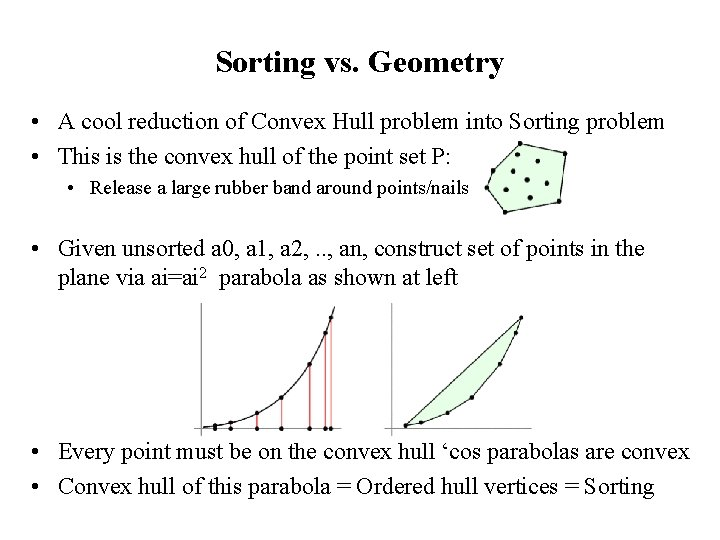Sorting vs. Geometry • A cool reduction of Convex Hull problem into Sorting problem • This is the convex hull of the point set P: • Release a large rubber band around points/nails • Given unsorted a 0, a 1, a 2, . . , an, construct set of points in the plane via ai=ai 2 parabola as shown at left • Every point must be on the convex hull ‘cos parabolas are convex • Convex hull of this parabola = Ordered hull vertices = Sorting